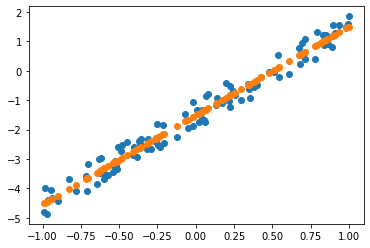# SGD from scratch

Mar 20, 2020 · 3 mins readShare this

## Stochastic Gradient Descent from scratch

learn = create_cnn(data, models.resnet34, metrics=error_rate, pretrained=Flase)


• Y=aX+b
• Y = a_1 X_1 + a_2 X_2 (X_2=1)
• a: coefficient(a_1: slope, a_2: intercept)
• X: parameter
• This is dot product - two thing multiplied and added
• Optimization
• loss.backward(): calculate the gradient
• a.sub_(lr * a.grad): take coefficient a, and substract gradient and multiply with learning rate, and substitute the value
• How gradient is calculated: The matrix calculus you need for deep learning
def update():
y_hat = x@a
loss = mse(y, y_hat)
if t % 10 == 0: print(loss)
loss.backward()
with torch.no_grad():
a.sub_(lr * a.grad)
a.grad.zero_()

• with torch.no_grad(): # turn gradient calculation off when you do sgd update
• at the real code, we make batch size, and slice some matrix (ex: y[:rand_idx]) and update the value.

Recap the terminology

• Learning rate: a thing that we multiply our gradient by, to decide how much to update the weights by
• Epoch: one complete run through all of our data points(highly related to overfitting)
• minibatch: random bunch of points that you use to update your weights
• SGD: gradient descent using minibatch
• Model / Architecture: kind of mean same thing. Architecture is the mathematical function that you’re fitting the parameters to.
• Parameter: Also known as coefficients, and also known as weights, are the number that you are updating.
• Loss function: the thing that’s telling you how far away or how close you are to correct answer

Bonus note

• When I was going to draw the prediction value, I got this error
---------------------------------------------------------------------------
RuntimeError                              Traceback (most recent call last)
<ipython-input-58-1650cee19828> in <module>()
1 plt.scatter(x[:, 0], y)
----> 2 plt.scatter(x[:,0], x@a)

7 frames
/usr/local/lib/python3.6/dist-packages/torch/tensor.py in __array__(self, dtype)
484     def __array__(self, dtype=None):
485         if dtype is None:
--> 486             return self.numpy()
487         else:
488             return self.numpy().astype(dtype, copy=False)

RuntimeError: Can't call numpy() on Variable that requires grad. Use var.detach().numpy() instead.


Regarding to pytorch forum, When I try to scatter it, it moves to numpy and meanwhile I will lose the gradient. so that I should detach() so that make Tensor does not requiring grad. And after that can move to numpy.